Next: Predictive distributions Up: Inferring numerical values of Previous: Inference from a data

## Multidimensional case -- Inferring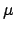and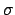of a Gaussian

So far we have only inferred one parameter of a model. The extension to many parameters is straightforward. Calling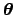the set of parameters and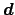the data, Bayes' theorem becomes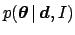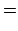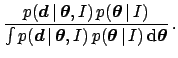(52)

Equation (52) gives the posterior for the full parameter vector. Marginalization (see Tab. 1) allows one to calculate the probability distribution for a single parameter, for example,, by integrating over the remaining parameters. The marginal distributionis then the complete result of the Bayesian inference on the parameter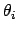. Though the characterization of the marginal is done in the usual way described in Sect. 5.1, there is often the interest to summarize some characters of the multi-dimensional posterior that are unavoidably lost in the marginalization (imagine marginalization as a kind of geometrical projection). Useful quantities are the covariances between parametersand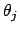, defined as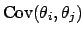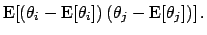(53)

As is well know, quantities which give a more intuitive idea of what is going on are the correlation coefficients, defined as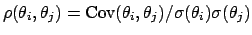. Variances and covariances form the covariance matrix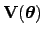, with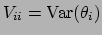and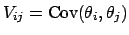. We recall also that convenient formulae to calculate variances and covariances are obtained from the expectation of the products, together with the expectations of the parameters: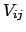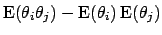(54)

As a first example of a multidimensional distribution from a data set, we can think, again, at the inference of the parameterof a Gaussian distribution, but in the case that alsois unknown and needs to be determined by the data. From Eqs. (52), (50) and (25), withand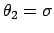and neglecting overall normalization, we obtain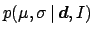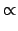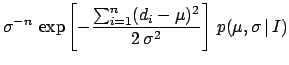(55)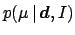(56)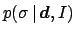(57)

The closed form of Eqs. (56) and 57) depends on the prior and, perhaps, for the most realistic choice of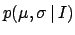, such a compact solution does not exists. But this is not an essential issue, given the present computational power. (For example, the shape ofcan be easily inspected by a modern graphical tool.) We want to stress here the conceptual simplicity of the Bayesian solution to the problem. [In the case the data set contains some more than a dozen of observations, a flat, with the constraint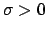, can be considered a good practical choice.]Next: Predictive distributions Up: Inferring numerical values of Previous: Inference from a data
Giulio D'Agostini 2003-05-13